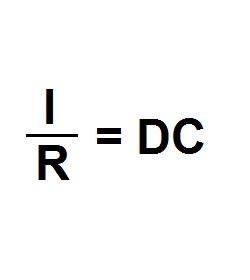Formula - Direct Capitalization | Propertylogy
Don't Miss

# Formula – Direct Capitalization

By on October 3, 2018Where:

I = Net operating income

R = Capitalization rate

DC = Direct capitalization

It is important to note that when direct capitalization is calculated, the assumption is that the cash flow is perpetual and the capitalization rate is constant which will remain the same.

If both assumptions are not present, then the discounted cash flow method will be a more dependable method of calculation.

Send this to a friend